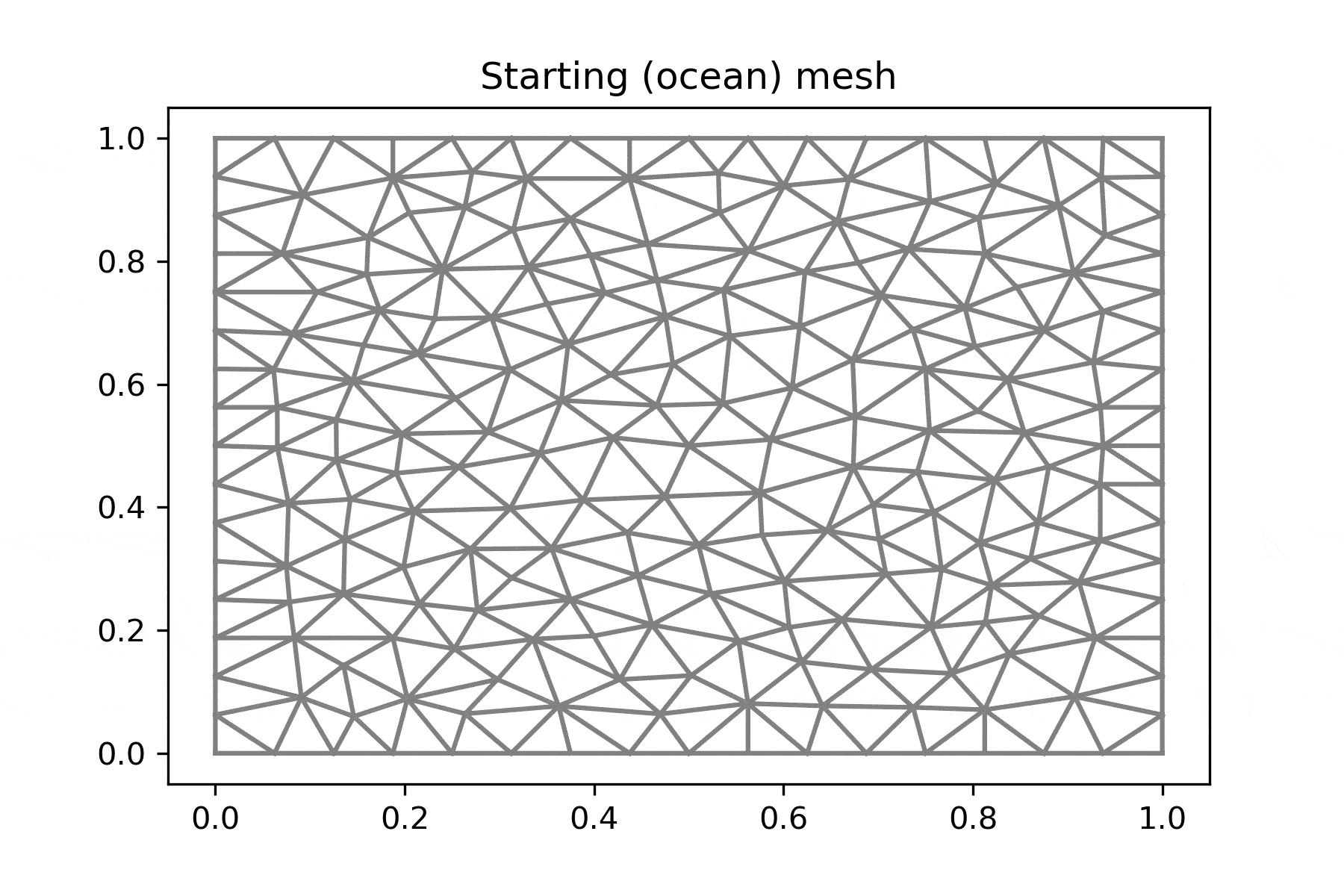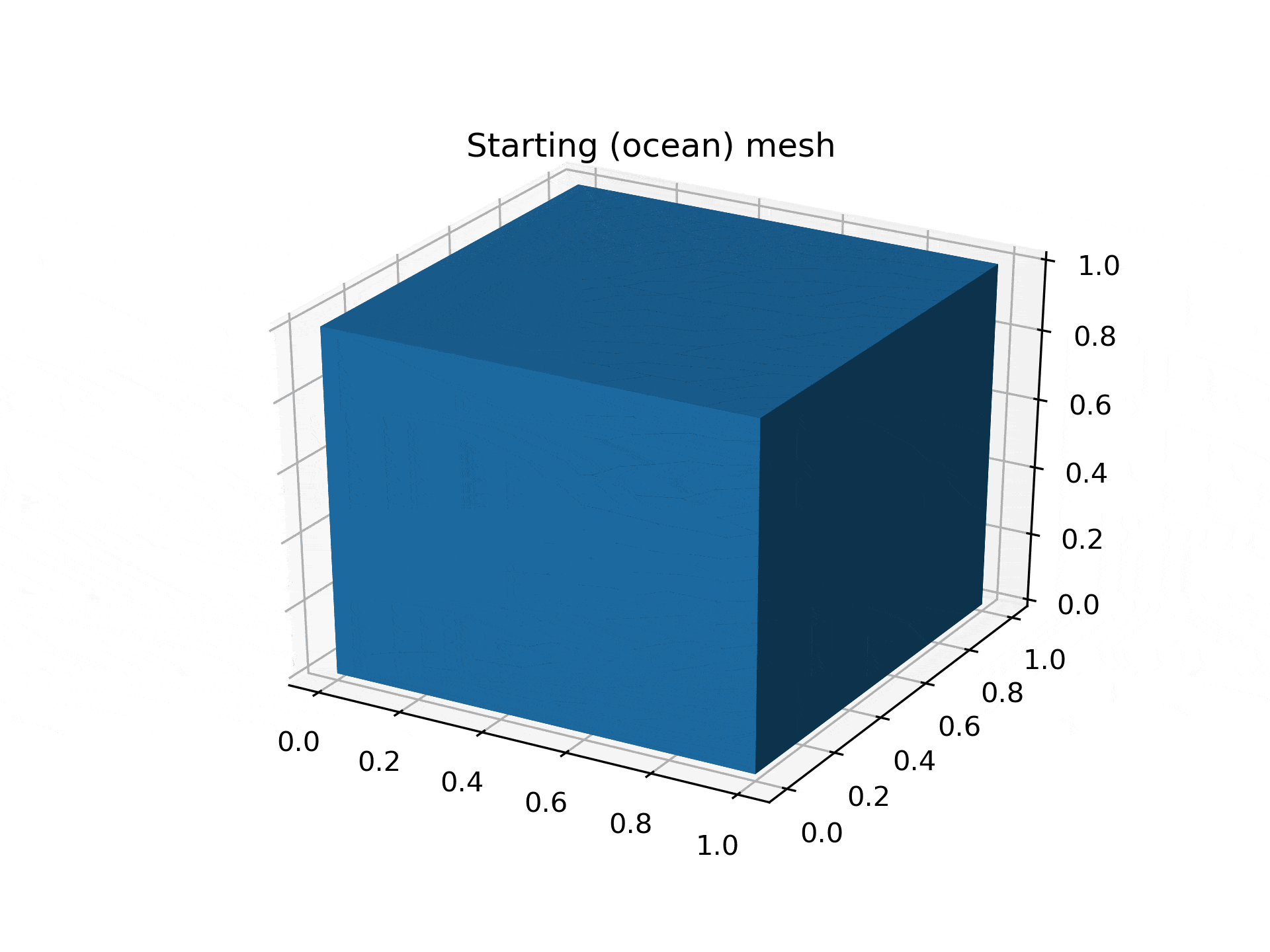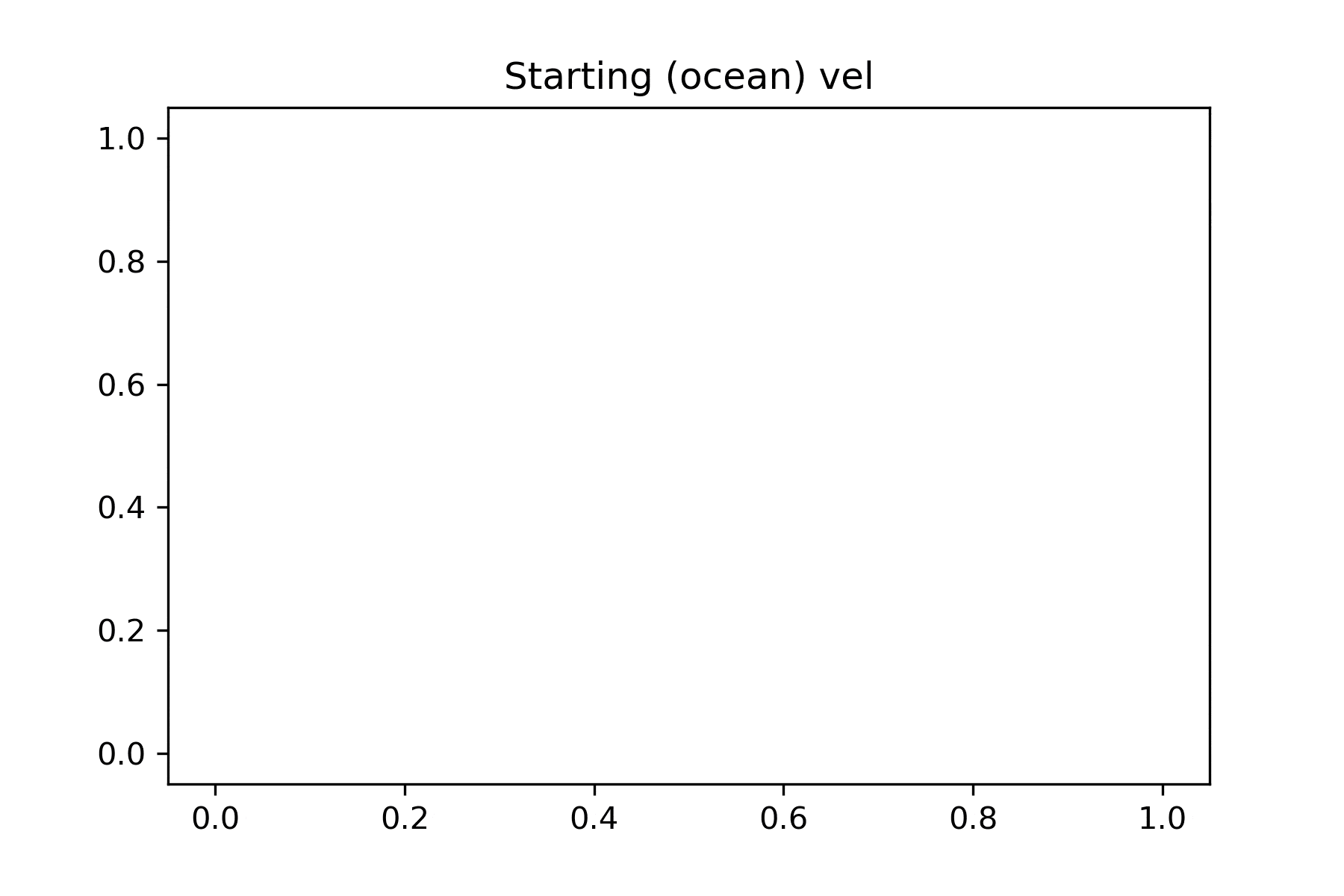# Deform an unstructured mesh (or how a good angle dramatically simplifies a problem)

Posted on Sat 23 April 2022 in programming, finite element method, mathematics

In the attempt of coupling my PhD's Finite Elements Framework for Fjord Ice-ocean Interactions (FEFFII) with an ice code, I stumbled upon the issue of deforming the ocean mesh. If a simulation stretches over a long span of time, the geometry of the ice is likely to change due to melting or re-freezing, so that the interface between the ice and the ocean can become different. If the ice melts, it gives way and leaves room, and that new space should be filled with "new" ocean. However, the ocean mesh cannot be dragged towards the ice through some simple transformation, because the new ice geometry can be quite irregular. If the mesh is instructured, the problem becomes even more complicated.

What follows is my end result, where a 2D unstructured mesh is deformed to match a new boundary layout. I will now detail how I first tackled the problem from the wrong angle, and then how I did solve it with a technique that generalizes to 3D meshes as well. The final algorithm is implemented as part of the FEFFII framework, in feffii.boundaries.deform_mesh.## A false start

At first, I thought that since the ocean and ice meshes had an exact common boundary at the beginning of the simulation, it should be possible to keep them in sync by monitoring the evolution of individual nodes, sort of. The idea was that, if the ice profiles changes a bit, I could check where the first node had moved towards, and adjust the first node of the ocean boundary accordingly. Repeating the same procedure for the second node, and then the third, and so on, and I could cover the whole boundary and move the ocean mesh to mimick how the ice had changed.

There were (at least) two issues with this approach:

• the ice mesh and ocean mesh could have different resolutions. This means that, while the first nodes of the two meshes would always match, the second node of the two meshes could have different coordinates, so I could not simply move the second node of the ocean mesh with respect to the second node of the ice mesh. This did not seem too much of a big deal: I could smoothly interpolate the change from two ice nodes and spread it over the many ocean nodes found in between.
• the meaning of first, second, third, etc, are straightforward on a line (the boundary of a 2D mesh), but lose any clear sense in two dimensions. What is the first point in the surface of a square? While there is no way of putting an ordering on a continuous 2D surface (theorem), it is possible to do so on a discrete surface, which is enough for any application. But this would require employing space-filling curves to estabilish a mapping between a line and a surface, blowing up the complexity of the project by an enourmous degree! (This point would have not mattered if I only cared about 2D meshes. However, since I was looking for a technique that would generalize to 3D meshes, I had to care.)

## A good solution

A different approach is order-agnostic, one that aims at moving each ocean boundary node without caring of where it is located. Intuitively, we can think of each point of the ocean boundary being pulled from its 2 or 3 closest neighbors with force proportional to their proximity. More formally, the idea is to scan the ocean boundary nodes and, for each node $$x$$,

• select $$k$$ closest neighbours among the ice boundary nodes, with $$k$$ = 2 or 3 depending on whether mesh is 2D or 3D;
• compute a mean of these closest neighbors, weighted (inversely) with respect to the distance from $$x$$;
• move $$x$$ to the newly-computed mean.

This procedure works both for 2D and 3D meshes and makes no assumptions on the structure of the mesh: all that is needed is a list containing the points of the new goal ice mesh. Since the mesh does not acquire nor lose any nodes, it is possible to deform it in this way and still solve PDEs on them that require time stepping.

What follows is a deformation example in 3D and a 2D example where the ocean velocity profile keeps evolving while the mesh changes over subsequent time steps.